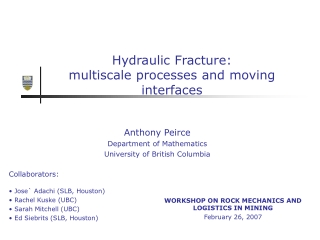DownloadDownload PresentationHydraulic Fracture: multiscale processes and moving interfaces

# Hydraulic Fracture: multiscale processes and moving interfaces

Télécharger la présentation## Hydraulic Fracture: multiscale processes and moving interfaces

- - - - - - - - - - - - - - - - - - - - - - - - - - - E N D - - - - - - - - - - - - - - - - - - - - - - - - - - -
##### Presentation Transcript

1. Hydraulic Fracture: multiscale processes and moving interfaces Anthony Peirce Department of Mathematics University of British Columbia • Collaborators: • Jose` Adachi (SLB, Houston) • Rachel Kuske (UBC) • Sarah Mitchell (UBC) • Ed Siebrits (SLB, Houston) WORKSHOP ON ROCK MECHANICS AND LOGISTICS IN MINING February 26, 2007

2. Outline • What is a hydraulic fracture? • Mathematical models of hydraulic fracture • Scaling and special solutions for 1-2D models • Numerical modeling for 2-3D problems • Conclusions

3. HF Examples - block caving

4. HF Example – caving (Jeffrey, CSIRO)

5. HF Examples – well stimulation

6. Model EQ 1: Conservation of mass

7. 1-2D model and physical processes Viscous energy loss Leak-off Fracture Energy Breaking rock

8. Scaling and dimensionless quantities • Rescale: • Dimensionless quantities: • Governing equations become:

9. Toughness dominated propagation • Asymptotic behaviour of the Hilbert transform • Large toughness:

10. Viscosity dominated propagation • Asymptotic behaviour of the Hilbert transform • Large viscosity:

11. HF experiment (Bunger& Jeffrey CSIRO)

12. 2-3D HF Equations • Elasticity • Lubrication • Boundary conditions at moving front (non-locality) (non-linearity) (free boundary)

13. Coupled equations – a model problem Elasticity Fluid Flow n-2 n-1 n n+1 n+2

14. Conditioning of the Jacobian • Evolution equation for w: • Eigenvalues of AC: Explicit Scheme Use implicit time stepping

15. General first order iterative method • Consider solving: using the iterative method • Residual errors are damped according to

16. Damping factor Poorly Damped Well Damped

17. Multigrid Methods

18. MG approach for coupled HF Equations

19. MG preconditioning of • C coarsening using dual mesh • Localized GS smoother

20. function [A] = lu(A) [n,n]=size(A); for j=1:n for k=1:j-1 for i=k+1:n A(i,j)=A(i,j)-A(i,k)*A(k,j); end end for i=j+1:n A(i,j)=A(i,j)/A(j,j); end end return function [A] = basicILUc(A) [n,n]=size(A); for j=1:n for k=1:j-1 if A(k,j) ~=0, for i=k+1:n if A(i,j) ~=0, A(i,j)=A(i,j)-A(i,k)*A(k,j); end end end end for i=j+1:n if A(i,j) ~=0, A(i,j)=A(i,j)/A(j,j); end end end return Incomplete LU factorization

21. Local Jacobian & Fourier Analysis Elasticity Fluid Flow n-2 n-1 n n+1 n+2

22. Spectrum of AC (ACLoc)-1

23. Eigenvalues of preconditioners

24. Numerical results for stress jump

25. Numerical results for stress jump

26. Front evolution via the VOF method 0.75 0.15 1.0 0.78 0.06 1.0 1.0 0.3

27. Time stepping and front evolution Time step loop: VOF loop: Coupled Solution end next VOF iteration next time step